# Three numbers

The sum of the three numbers is 287. These numbers are in a ratio of 3: 7: 1/4. Find these numbers.

a =  84
b =  196
c =  7

### Step-by-step explanation: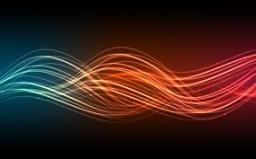Did you find an error or inaccuracy? Feel free to write us. Thank you!Tips to related online calculators
Check out our ratio calculator.

## Related math problems and questions:

• Find the sumFind the sum of all natural numbers from 1 and 100, which are divisible by 2 or 5
• Sum and ratioThe sum and ratio of two numbers is equal to 10. Which numbers are they?
• Sum of odd numbersFind the sum of all odd integers from 13 to 781.
• Pennies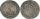800 pennies have the same value as 100 ducats. 100 pennies have the same value as 250 tolars. How many ducats has the same value as 100 tolars?
• Division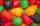Three siblings Helena, Oliver and George split the bag with candies on merit in the ratio 6:1:4. How many candies should each get if in bag were 88?
• Ratio three numbers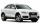Three numbers SUV are in the ratio 1:2:3. Their sum is 24. Find this numbers and write their add and sum.
• In theIn the national park, the ratio of the wooded area to grassland is 4: 1. The total area is 385km2. What area is wooded?
• Ratio6 numbers are in the ratio 1:5:1:5:5:5. Their sum is 242. What are the numbers?
• ŽSRCalculate fixed annual personnel costs of operating monorail line 118 km long if every 5 km is a station, which serves three people - one dispatcher and two switchmen in 4-shift operation. Consider the average salary of the employee 885 €.
• Probably memberLook at the series 2,6,25,96,285, ? What number should come next?
• Sum of the seventeen numbersThe sum of the 17 different natural numbers is 154. Determine the sum of the two largest ones.
• Numbers at ratio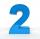The two numbers are in a ratio 3:2. If we each increase by 5 would be at a ratio of 4:3. What is the sum of original numbers?
• CuboidThe sum of the lengths of the three edges of the cuboid that originate from one vertex is 210 cm. Edge length ratio is 7: 5: 3. Calculate the length of the edges.
• Gear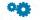Two gears, fit into each other, has transfer 2:3. Centres of gears are spaced 82 cm. What are the radii of the gears?
• Sides ratioCalculate the circumference of a triangle with area 84 cm2 if a:b:c = 10:17:21
• Divide 5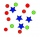Divide 288 in the following ratio 3 : 4 : 5
• Chicks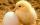How many chicks were hatched from 4500 eggs, when an average of 100 eggs hatched 87 chicks?